# Column vectors

We know a vector can be represented by a line which has magnitude (how long it is) and direction.

In graphical vectors, this is represented by: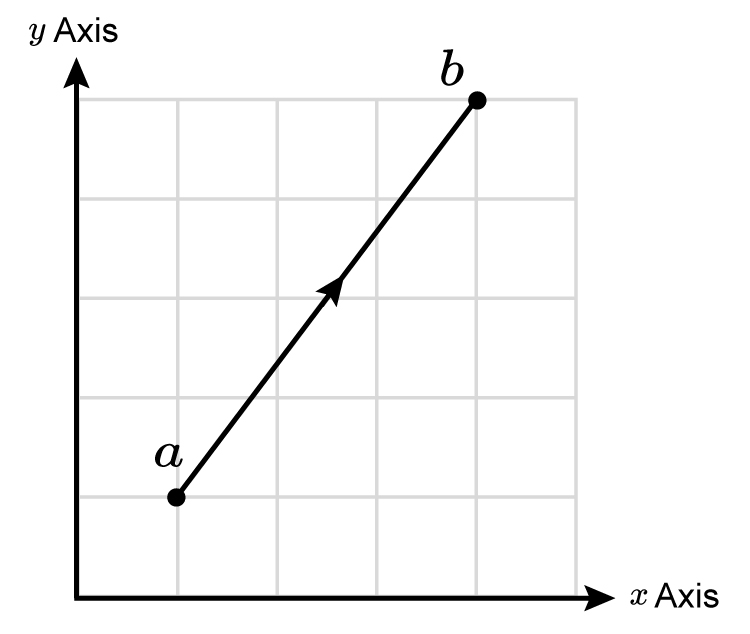or as a column vector ((3),(4))

That is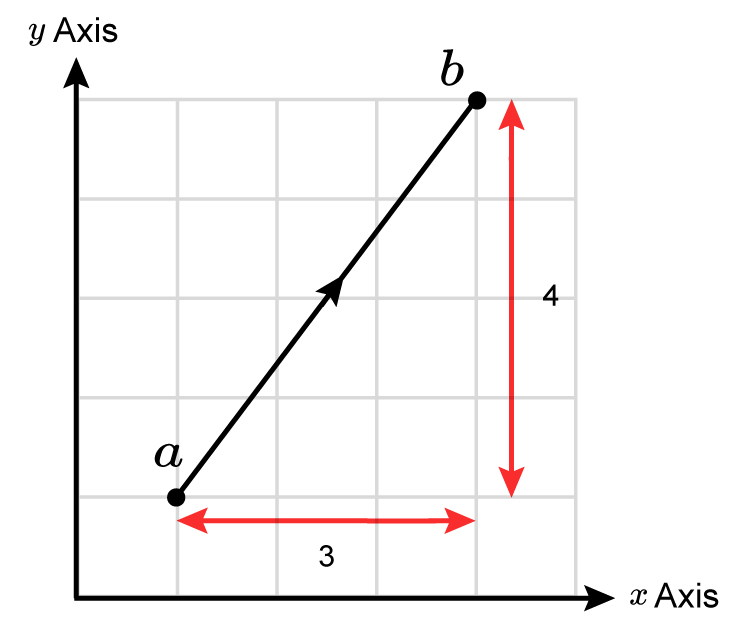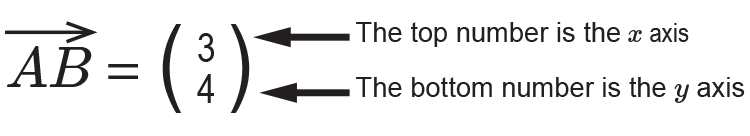NOTE:

(Do not get confused between coordinates (3,4) which is the position of a point x  along and y  up from zero).

In column vectors, the numbers represent a distance and direction from any point.

In column vectors x  is on the top and y  is on the bottom. For coordinates we have:

Coordinates are written alphabetically
so X comes before Y (X,Y).

But in column vectors so does x come before y.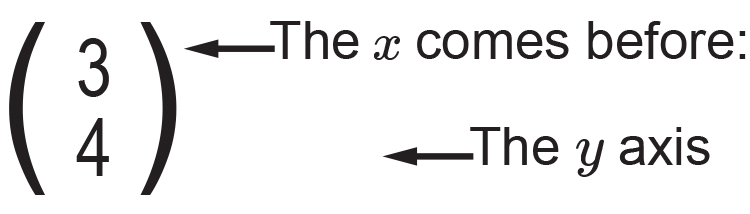Example 1

Convert the vector vec (AB)  to a column vector.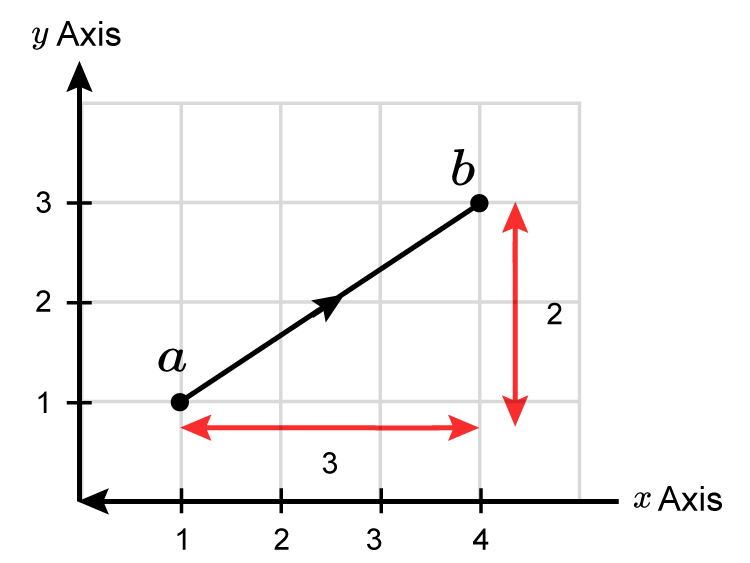Vector vec (AB)=((3),(2))